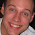# Jeromy Anglim's Blog: Psychology and Statistics

## Friday, September 25, 2009

### Discriminant Function Analysis in a Nutshell | Overview, Alternatives, and Resources

This post discusses Discriminant Function Analysis (DFA). It sets out the basic purpose of DFA and provides some links to additional resources.

DFA in a nutshell:
The most common scenario for DFA that I encounter (note, it's not the only DFA scenario) is the following: a) one categorical dependent variable (DV); two or more (typically 4 or more) numeric independent variables (IVs). DFA forms a composite of the IVs which maximises prediction of the DV. The researcher's aim is typically to 1) see whether the predictors can be used to correctly predict (classify) the DV; and 2) see how the predictors are related to the composite.
Some other options:

• Logistic regression: If the DV is binary, you can use binary logistic regression; If the DV has 3 or more unordered categories, you can use multinominal logistic regression. In terms of choosing between logistic regression and DFA, many researchers prefer logistic regression because it makes fewer assumptions (see a book like Tabachnick and Fidell for further discussion).
• Optimal Scaling Regression (Catreg in SPSS): Although I've never checked, I'd imagine you'd get similar results with optimal setting a nominal measurement model for your DV and numeric for the IVs. Optimal scaling is more exploratory and provides greater freedom. However, this freedom can lead to less comparability between studies and over fitting to the sample, if you're not careful.
If you are making a decision between the various options, have a read through some references and examine the pros and cons and remember the basic rule of statistical analysis, if you do it both ways and it doesn't make a difference, then it doesn't really matter.

References

1.hi
tnx for your post
do u have any MatLab code or another programming languages about : discriminant function analysis
I would be appreciated you if you help me about the codes...my email is:mrebrahimi709@gmail.com
best regard for you

2.3.